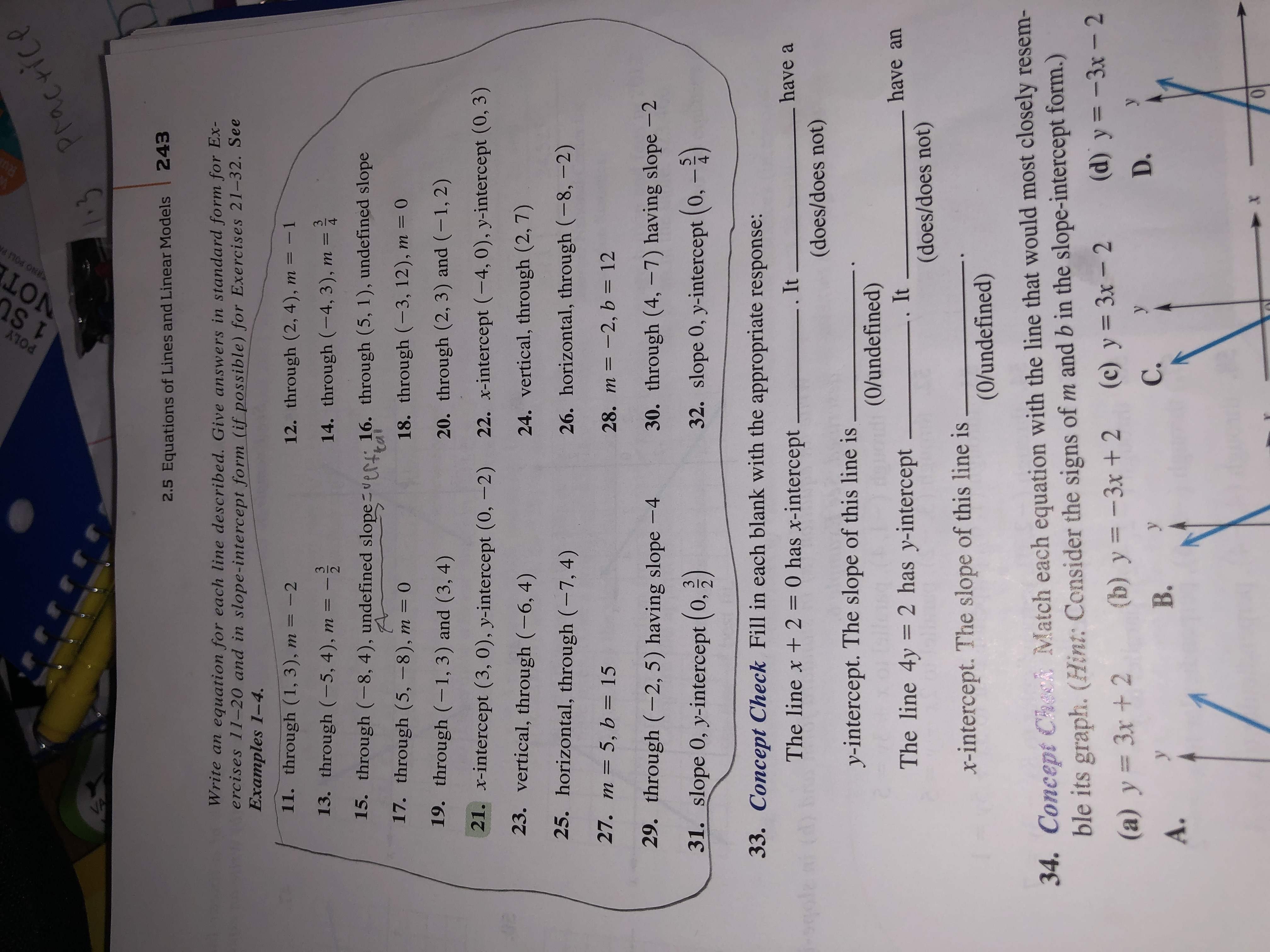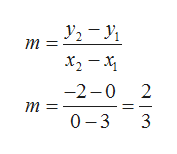# RUrrrrPracrice2.5 Equations of Lines and Linear Models 243Write an equation for each line described. Give answers in standard form for Ex-ercises 11-20 and in slope- intercept form (if possible) for Exercises 21-32. SeeExamples 1-4.11. through (1, 3), m = -212. through (2, 4), m = -1313. through (-5, 4), m =14. through (-4, 3), m =2415. through (-8, 4), undefined slope er 16. through (5, 1), undefined slopetai17. through (5, -8), m = 018. through (-3, 12), m = 019. through (-1, 3) and (3, 4)20. through (2, 3) and (-1, 2)21. x-intercept (3, 0), y-intercept (0,-2)22. x-intercept (-4, 0), y-intercept (0, 3)0323. vertical, through (-6, 4)24. vertical, through (2, 7)25. horizontal, through (-7, 4)26. horizontal, through (-8,-2)27. m 5, b = 1528. m -2, b = 1229. through (-2, 5) having slope -430. through (4, -7) having slope -231. slope 0, y-intercept (0, 2)32. slope 0, y-intercept (0,-233. Concept Check Fill in each blank with the appropriate response:The line x + 2 = 0 has x-interceptIthave(does/does not)y-intercept. The slope of this line is(0/undefined)The line 4y 2 has y-intercept_have anIt(does/does not)x-intercept. The slope of this line is(0/undefined)34. Concept Check Match each equation with the line that would most closely resem-ble its graph. (Hint: Consider the signs of m and b in the slope-intercept form.)(b) y-3x+ 2(d) y-3x - 2(c) y 3x- 2(a) y 3x+ 2уD.С.В.А.POLY1 StVOT141Cs omEs

Question

21: Write in slope-intercept form.

How do you proceed?help_outlineImage TranscriptioncloseRU rrrr Pracrice 2.5 Equations of Lines and Linear Models 243 Write an equation for each line described. Give answers in standard form for Ex- ercises 11-20 and in slope- intercept form (if possible) for Exercises 21-32. See Examples 1-4. 11. through (1, 3), m = -2 12. through (2, 4), m = -1 3 13. through (-5, 4), m = 14. through (-4, 3), m = 2 4 15. through (-8, 4), undefined slope er 16. through (5, 1), undefined slope tai 17. through (5, -8), m = 0 18. through (-3, 12), m = 0 19. through (-1, 3) and (3, 4) 20. through (2, 3) and (-1, 2) 21. x-intercept (3, 0), y-intercept (0,-2) 22. x-intercept (-4, 0), y-intercept (0, 3) 03 23. vertical, through (-6, 4) 24. vertical, through (2, 7) 25. horizontal, through (-7, 4) 26. horizontal, through (-8,-2) 27. m 5, b = 15 28. m -2, b = 12 29. through (-2, 5) having slope -4 30. through (4, -7) having slope -2 31. slope 0, y-intercept (0, 2) 32. slope 0, y-intercept (0,-2 33. Concept Check Fill in each blank with the appropriate response: The line x + 2 = 0 has x-intercept It have (does/does not) y-intercept. The slope of this line is (0/undefined) The line 4y 2 has y-intercept_ have an It (does/does not) x-intercept. The slope of this line is (0/undefined) 34. Concept Check Match each equation with the line that would most closely resem- ble its graph. (Hint: Consider the signs of m and b in the slope-intercept form.) (b) y-3x+ 2 (d) y-3x - 2 (c) y 3x- 2 (a) y 3x+ 2 у D. С. В. А. POLY 1 St VOT 141Cs omEs fullscreen
check_circle

Step 1

Since x-intercept is (3,0) and y-intercept is (0,-2), therefore , the line passes through the points (3,0) and (0,-2).

We know that slope of line passing through the points (x1,y1) and (x2,y2) ishelp_outlineImage TranscriptioncloseУ, — У, т 3. х, — х -2-0 2 т 3D 0-3 3 fullscreen
Step 2

The slope - intercept form of line is y=mx+c

He...

### Want to see the full answer?

See Solution

#### Want to see this answer and more?

Solutions are written by subject experts who are available 24/7. Questions are typically answered within 1 hour.*

See Solution
*Response times may vary by subject and question.
Tagged in

### Equations and In-equations